Bottom line: Learn how to write an IF formula in Excel using the IF function.

Skill level: Beginner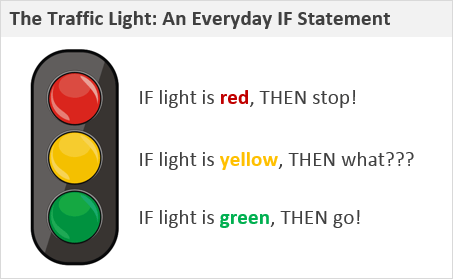## Everything You Do Is An IF Statement

Everyday we do hundreds (if not thousands) of IF statements in our head. Every decision we make is an IF statement:

• IF I eat breakfast, THEN I will have energy for work/play.
• IF I do the laundry, THEN my wife will be happy.
• IF I brush my teeth, THEN more people will talk to me.

My favorite example is the old traffic light analogy.

• IF the light is green, THEN go.
• IF the light is red, THEN stop.
• IF the light is yellow, THEN what???  We'll talk about that a little later…

These IF statements are also referred to as logical tests.  We are testing for a condition (the color of the light), then taking an action based on whether the condition is TRUE or FALSE.

Writing your first IF formula in Excel is an exciting moment.  It gives you the feeling of how you can program Excel to make it interactive.

## Logical Tests – You Don't Always Need the IF Function

Before you write your first IF statement (formula), it's best to get an understanding of how Excel makes comparisons.

Type your first name in cell A1 and your last name in cell B1.  Then type the following formula in cell C1:

=A1=B1

If your first name and last name are different, then the result will be FALSE.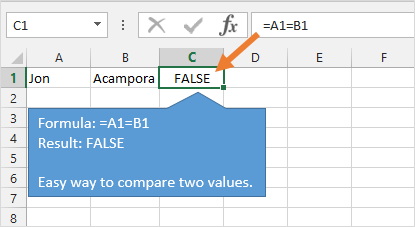Now type the number 2 in cell A2 and B2.  Then type the following formula in cell C2:

=A2=B2

The result will be TRUE.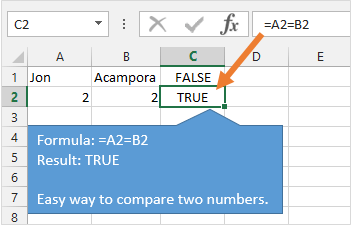We are using the equal sign “=” to compare the two values.  The result will always be TRUE or FALSE (unless one of the cells contains an error).

This also works with the greater than “>” and less than “<” symbols.  These are called comparison operators.

### Comparison Operators in Excel

 Operator Description = Equal To <> Not Equal To > Greater Than < Less Than >= Greater Than or Equal To <= Less Than or Equal To

Using the comparison operators in a formula is a very EASY way to compare two values in Excel.  It's a great way to compare lists of names, text, dates, or numbers.

It's also important to note that the case doesn't matter when comparing text.  The following formula will return TRUE.

=”Excel Campus” = “excel campus”

## The IF Function Explained

What if you want to return a result other than TRUE or FALSE?

For this we can use Excel's IF function.

`=IF(logical_test, value_if_true, [value_if_false])`

The IF function is pretty simple, and contains the following three arguments.

1. Logical Test
2. Value If True
3. Value If False (optional)For the 1st argument, Logical Test, we can use the same formula we used above to compare two values.

If the logical test evaluates to TRUE, then the result of the IF function will return whatever you put in the 2nd argument (Value If True).

The Value If True argument can contain just about anything:

• A number
• Text wrapped in double quotes – “hello”
• A reference to another cell – A2
• A formula with another IF function.
• A formula with any other combination of functions.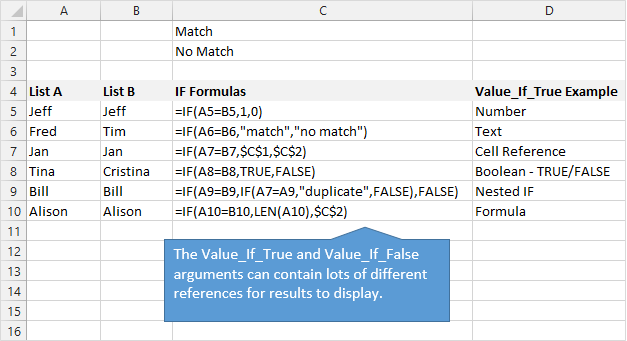The 3rd argument, Value If False, will be returned if the Logical Test (1st argument) evaluates to FALSE.  It can hold any of the same items that the Value if True argument can hold.

The 3rd argument is Optional.  This means that you don't have to put anything in the formula for it.  You can just close the parenthesis after the 2nd argument.  If the logical test is false then the formula result will display FALSE in the cell.

## Nested IF Formulas – Yellow Light!!!

So we can see how to program our decision for the green light and red light, but what if the light is yellow???

This is where we can get in trouble in life (and Excel).

You have a decision to make, and you have to make it fast!  Your brain does a series of logical tests within a second or two.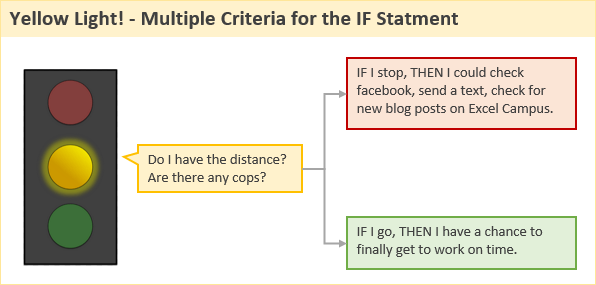The first test is probably, “Do I have enough distance to stop?”  Then, “Are there any cops around?”

Then it's something like, “If I stop I definitely won't risk anything.  Plus, I could check Facebook, send that text, or see if there are any new blog posts on Excel Campus…”

“If I go, then I'll have a chance to finally get to work on time…”

It's never an easy decision.  But more importantly, how would we write a formula for this decision?

One way is to use a bunch of IF functions in the same formula.  This is called a nested IF formula.  As you can see in the image below, the formula can get long and ugly.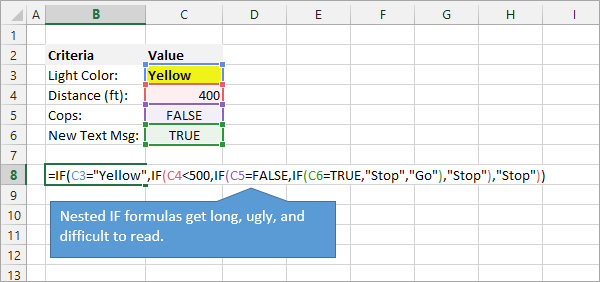The nested IF formula also becomes difficult to read and interpret.

Fortunately, there are lots of alternatives to nested IF formulas in Excel.  We can use:

• Lookup functions like VLOOKUP, INDEX, MATCH
• Logical functions like CHOOSE, AND, OR, NOT
• Math functions like SUMIFS, COUNTIFS, AGGREGATE

Each of these alternatives require an explanation, and I will write separate articles for those.  They can be much more efficient to read, write, and calculate.

Check out my free video training series on the lookup functions to learn more.

## Programming Excel with the IF Function

Writing IF formulas gives us a powerful feeling with Excel.  You start to see how you can use it to display results based on criteria or user inputs.  This can make your financial models, charts, and dashboards interactive.

You are basically programming Excel with formulas to build spreadsheet applications.  If you are interested in programming and automating Excel even further, checkout my free video training series on Macros and VBA.

•ping says:

in a long list of a shipping data, I need to find instances of a Part Number with dates in column A and qty in column C, a function to select the latest date and then total all qty listed in column C. It seems like an easy VLookup function, I’d hate to write a VBA for something like this. Is there a simple and elegant x/vlookup function for this?

•play golf says:

Hello, you used to write fantastic, but the last several posts have been kinda
boring… I miss your super writings. Past few posts are just a little out of track!
come on!

•Barbara says:

Just incredible your tutorials. Your tutorials are so well thought out and clear to understand and follow. Your tutorials have helped me so much in my new job for analysing data. Thanks just does not say it, how grateful I am that you have done these tutorials.

•QuickBooks Error 80029c4a says:

I truly enjoy looking at on this web site, it has got good blog posts.

•Deborah says:

whoah this weblog is fantastic i really
like studying your articles. Keep up the great work!
You recognize, many persons are hunting round for this info, you can aid them greatly.

•bokep jepang says:

I like this website because so much utile material on here :D.

•Brendan says:

Keep up the great work! You understand, many individuals are hunting around for this info, you can aid them greatly.

•Sherrill says:

Why people still use to read news papers when in this technological world the whole thing is presented
on net?

•Beverly says:

I have read so many content on the topic of the blogger
lovers but this paragraph is truly a nice paragraph, keep it up.

•David says:

Hi, I would like to have a cell reference another with an IF statement like this:

IF the value of any Cell in Column A of Sheet 1 is 100 then this cell (Sheet 2, cell A2 for example) should be the value of Column D for the row where the value of the Cell in Column A is 100. And also Column D for that Row may change whereas it’s Column A value for that same Row may stay the same.

This means i want to look for changes in Column A. Sometimes 100 might be Row 2, but sometimes it might be Row 302. Also, if Sheet 1, Column A, Value=100 stays in the same Row, but its Column D value changes, I’d want Sheet 2, Cell A2 to reflect that change in Column D for the Row where Value=100. And I want Sheet 2, Cell A2 to reflect whatever Column D is for the row where Column A equals 100.

This seems kind of complicated, and I’m really trying to make sense of the logic for myself too, but ANY direction or advice would be sooooo appreciated. Thanks, Excel warriors!

•Konstantin says:

Thank you for the helpful article.
I am experiencing an unexpected difficulty that I cannot find the solution to.
The return values are being returned as text. But what I am trying to do is to have the return values be numbers which I can subsequently sum up. Is there a way to make the return value be a number that excel recognizes as a number instead of a text

•Roxanne says:

I am trying to write a formula for when the sum in B67 is greater than -5% then JOB PERFORMANCE ANALYSIS REQUIRED is displayed in cell A71.

Thanks so much for any assistance you can provide.

•Alexandra Kovalenko says:

Hello, I am trying to create a formula to have different calculations on specific day of the week. On weekdays value should just be a value of another cell multiplied by 0.85 (X*0.85), and on weekends it should be a value of another call multiplied by 0.85 minus 10 (X*0.85-10). Can anybody recommend how to build this formula?

•vishnu says:

Write a function is_older that takes two dates and evaluates to true or false. It evaluates to true if
the First argument is a date that comes before the second argument. (If the two dates are the same,
the result is false.)

•PAIGE says:

HEY THIS IS GREAT STUFF! I’m trying to write statements that compare three piece of data
Date compared to another date, age and length of stay. There are three variables and 12 possible answers and I know there is a good way to do this with nested IF statements, IS_Before date tests but!~ please help!

IF date is before “hard date” and age <=14, and {staying} = "weekend" then result = \$200
IF date is before "later hard date" and age <=14, and {staying} = "weekend" then result = \$250
IF date is before "last hard date" and age <=14, and {staying} = "weekend" then result = \$300

IF date is before "hard date" and age <=14, and {staying} = "week" then result = \$375
IF date is before "later hard date" and age <=14, and {staying} = "week" then result = \$450
IF date is before "last hard date" and age 14, and {staying} = “weekend” then result = \$325
IF date is before “later hard date” and age >14, and {staying} = “weekend” then result = \$375
IF date is before “last hard date” and age >14, and {staying} = “weekend” then result = \$450

IF date is before “hard date” and age >14, and {staying} = “week” then result = \$425
IF date is before “later hard date” and age >14, and {staying} = “week” then result = \$500
IF date is before “last hard date” and age >14, and {staying} = “week” then result = \$575

•Jon Acampora says:

Hi Paige,

The best way to solve this is probably with a SUMIFS or SUMPRODUCT formula. You could use a nested IF formula, but that formula would be very long and difficult to understand/modify.

With SUMIFS or SUMPRODUCT you could build a table with all the conditions, then have the formula evaluate each condition against the data for each person.

I don’t believe I have any article that cover this type of formula with multiple conditions yet, but I will add it to the list for the future.

I hope that helps. Thanks!

•Laura says:

How do i write a formula which calculates if there is a 44 in column g only if there is a number in column m?

•Gio says:

What formula can I create to make a cell turn a certain color based on data in another cell? For example, If in cell C3 is the word “Yes” then I want cell D3 to be the color Yellow. Thanks.

•Shirley says:

Hi,

I have staff reports I’m trying to merge so I can create a pivot table for the data. The issue is the staff names are configured differently in each report (ie. JSMITH on one report and Smith, John on another). Is there a formula I can use to say JSMITH = Smith, John so all names convert to one configuration?

•Nelson says:

IF formula can’t seem to work IF(Q3=”CLOSE”,O4=N4). Whenever Q3:Q1000 cells has the text “CLOSE”, the formula in cell N4 will automatically change from O4+N4 to O4=N4.

•Wanda says:

You have some great education here. I need a little more help. I have a cell with a date, I want in another cell to say if the date plus 365 days is older than today’s date, then Inspect, If not older than today’s day, then ok. I am doing something wrong. I came up with =IF((K3+365)<(today),"INSPECT","OK") but it doesn't seem to be working. Can you help?

•Jon Acampora says:

Hi Wanda,
The TODAY function just needs to have the parenthesesafter it, like the following.

`=IF((K3+365)The TODAY function does not require any arguments, so we don't have to specify anything between the parentheses.  However, it is referenced just like all other functions with arguments.  I hope that helps.`
•Charles says:

Hi Jon

I am trying to display one of 3 values for one of 3 answers
input display (“on another sheet”)
H = “half” 500
F = “full” 1000
L = “left” 0

so my input in one cell can be H or F or L and from the input determine

Sorta something like this “Doesn’t work though”
cell {(B1)}IF (A1=”H”),500; IF(A1=”F”),1000; IF(A1=”L”),0

How can I make this work

•Casey says:

I have two columns of information I would like to compare to create a value in a third column. For example, a person’s salary and the type of medical coverage they get. Say the employee makes \$49000 and has family coverage, I would like the third column to input a value based on the salary and coverage selected. The value will be based off of a predetermined premium sharing calculation that correlates the employees salary and coverage type. For example, \$45,000 to \$49,999 in salary and family coverage is equal to 14%.

•Myint Zaw says:

•Stefanie says:

I have a table with percentages in column 1, and dollar amounts in row 1. I am trying to write a formula that returns the value in the intersecting cells. Note – the dollar amounts in row one will be a range. Ex – Greater than or equal to \$0, and less than or equal to \$50,000. Any help is much appreciated!! I’m stumped!

•bac nho says:

I’m impressed, I mսst say. Seldom ⅾo I come ɑcross a
blog tһat’s bоth educative аnd engaging, and let mе teⅼl yߋu,
yⲟu’ve hit tһe nail on thе head. The issue іs an issue that not enoսgh people ɑrｅ speaking intelligently ɑbout.
Now i’m vｅry һappy that I stumbled across this in my hunt
for somеthing concerning this.

•Jackson says:

Hi,Jon
I am utterly confused with these few question below, would you please assist me?

If then, your help is much appreciated

Question:
Given an employees’s old salary in A10, formulate an IF statement that calculates a new salary based on the following;

an increase by the percentage value in A8 if the old salary is at most K2000, a decrease by the percentage value in A9 if the old salary is at least K3000 otherwise, the salary remains unchanged.

Thank you.

•Joseph says:

So I have written an IF statement, but the form is constantly updating. How can I write my IF statement to hold the last true value as the displayed value whenever it is False?

•Yana says:

Hi.. I want to write an excel formula for this:
1. If value 24= High

•Mark says:

Hi,

I need to update the following formula that check if the third character is X

=IF(LEFT(RIGHT(C2,LEN(C2)-2),1)=”X”,

What I need to change it to is to check if third character is X or Y before continue to the next IF formula

•Rinalyn says:

Im trying to make a formula on this:
If a cell(C5) is equal to 15,then the value is 15 however if the cell (C5) is less than 15, then I need to add cell(D5) to cell(C5) but the answer shoul be 15.

situation 1: cell(C5) = 6 ; cell(d5) = 23
situation 2: cell(c5) = 1 ; cell(d5) = 4

•ratha says:

Hi Jon Acampora
i want how to calculate amount add 5% in function if
i don’t know how write down this code

•Benny says:

Hi Jon
I am trying to figure this one out, so I can write a formula.
If the field C1 contains the value between 1 an 4, return “Peasant, else if C1 is between 5 and 9, return “Squire(ss), else if C1 is between 10 and 24, return “Sir/Dame”, else if C1 is between 25 and 49…. and so on, up to 10, that says if C1 is >999, return “Prince”.
How can I make this?

•J says:

The best way to do this (in my opinion) is with a vLookup formula. Create a table with the lower edge of the range and its matching item:

1 Peasant
5 Squire(ss)
10 Sir/Dame
etc.

Format it as a table and name the table something like tblTitles.

Then use =vlookup(C1,tblTitles,2) as your formula.

(This will look up the value of C1 in tblTitles and return the value from column 2 of that table.)

•Tanya says:

I am trying to write a formula for the following:

If J28 = 3% and is less than 5% the multiply J22 by 1.25; if not the “N/A”

this is the formula I used, and J28 = 3.9%, however, N/A is the result:

=IF((AND(J28=3%,J28<5%)),J22*1.25,"N/A")

•Mohsen says:

Hi Tanya,

You can change formula to this:
=IF(5%>J28>=3%,J22*1.25,”N/A”)
OR
=IF(0.05>J28>=0.03,J22*1.25,”N/A”)

•Roger says:

I need a formula where if the value of a cell is greater than/equal to the value of a second cell the answer is “above threshold”. And vice versa for “below threshold”.

•Jon Acampora says:

Hi Roger,
The following should work. The formula compares the value is cell A2 to see if it is greater than or equal to the value in cell B2.
=IF(A2>=B2,”above threshold”,”below threshold”)

•Zion says:

I think this question may relate to this?

How about if one column is greater than another for example if F3>G3 Copy the entire row A3 thru G3 and paste to sheet 2?

•Jon Acampora says:

Hi Zion,

You could use a macro for that. If you are new to macros, checkout my free 3-part video series on macros & VBA. I hope that helps.

•Niall says:

The is the best explanation I found.

Can I ask a question on an expansion on this??

I have this working great when used to search a single column but I would like to know how to make this work if A1 had to match a within a range.

What I use is:
=IF(\$B\$3=’External Audit’!\$F\$1,IF(F4=’External Audit’!A3,IF(‘External Audit’!F3=”EA”,”*”,””)))

What I would like to do is if A1 is September, It can search the headings for September, then match the row with what I have in F4, and get a value * or – returned if the cell contains EA.

Thanks
Niall

•Jon Acampora says:

Hi Niall,
Happy to hear you enjoyed it. I believe you can use the MATCH function instead of nested IF formulas. Here is an article that explains how to use the MATCH function in more detail. I also have a free 3-part video series on the lookup formulas including VLOOKUP and INDEX/MATCH. I hope that helps. Thanks again! 🙂

•just started says:

great work man ,,, thank you so much, it is so useful

•Amit says:

Very very interesting example.

•Debjit says:

great

•Adam says:

Neat explanation.
With many examples and a touch of humor

Generic filters
Exact matches only
Filter by Custom Post TypeLearn 10 great Excel techniques that will wow your boss and make your co-workers say, "how did you do that??"